## RD Sharma Class 9 Solutions Chapter 10 Congruent Triangles MCQS

These Solutions are part of RD Sharma Class 9 Solutions. Here we have given RD Sharma Class 9 Solutions Chapter 10 Congruent Triangles MCQS

Other Exercises

Mark the correct alternative in each of the following:
Question 1.
One angle is equal to three times its supplement. The measure of the angle is
(a) 130°
(b) 135°
(c) 90°
(d) 120°
Solution:
Let required angle = x
Then its supplement = (180° – x)
x = 3(180° – x) = 540° – 3x
⇒ x + 3x = 540°
⇒ 4x = 540°
⇒ x = $$\frac { { 540 }^{ \circ } }{ 4 }$$  = 135°
∴ Required angle = 135° (b)

Question 2.
Two straight lines AB and CD intersect one another at the point O. If ∠AOC + ∠COB + ∠BOD = 274°, then ∠AOD =
(a) 86°
(b) 90°
(c) 94°
(d) 137°
Solution:
Sum of angles at a point O = 360°
Sum of three angles ∠AOC + ∠COB + ∠BOD = 274°∴ Fourth angle ∠AOD = 360° – 274°
= 86° (a)

Question 3.
Two straight lines AB and CD cut each other at O. If ∠BOD = 63°, then ∠BOC =
(a) 63°
(b) 117°
(c) 17°
(d) 153°
Solution:
CD is a line
∴ ∠BOD + ∠BOC = 180° (Linear pair)⇒ 63° + ∠BOC = 180°
⇒ ∠BOC = 180° – 63°
∴ ∠BOC =117° (b)

Question 4.
Consider the following statements:
When two straight lines intersect:
(iii) opposite angles are equal
(iv) opposite angles are supplementary Of these statements
(a) (i) and (iii) are correct
(b) (ii) and (iii) are correct
(c) (i) and (iv) are correct
(d) (ii) and (iv) are correct
Solution:
Only (ii) and (iii) arc true. (b)

Question 5.
Given ∠POR = 3x and ∠QOR = 2x + 10°. If POQ is a striaght line, then the value of x is
(a) 30°
(b) 34°
(c) 36°
(d) none of these
Solution:
∵ POQ is a straight line
∴ ∠POR + ∠QOR = 180° (Linear pair)
⇒ 3x + 2x + 10° = 180°
⇒ 5x = 180 – 10° = 170°
∴ x = $$\frac { { 170 }^{ \circ } }{ 5 }$$  = 34° (b)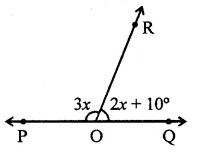Question 6.
In the figure, AOB is a straight line. If ∠AOC + ∠BOD = 85°, then ∠COD =
(a) 85°
(b) 90°
(c) 95°
(d) 100°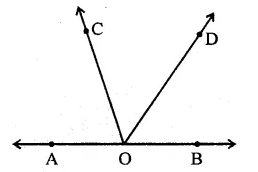Solution:
AOB is a straight line,
OC and OD are rays on it
and ∠AOC + ∠BOD = 85°
But ∠AOC + ∠BOD + ∠COD = 180°
⇒ 85° + ∠COD = 180°
∠COD = 180° – 85° = 95° (c)

Question 7.
In the figure, the value of y is
(a) 20°
(b) 30°
(c) 45°
(d) 60°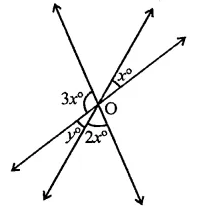Solution:
In the figure,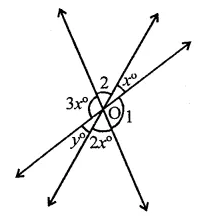y = x (Vertically opposite angles)
∠1 = 3x
∠2 = 3x
∴ 2(x + 3x + 2x) = 360° (Angles at a point)
2x + 6x + 4x = 360°
12x = 360° ⇒ x = $$\frac { { 360 }^{ \circ } }{ 12 }$$  = 30°
∴ y = x = 30° (b)

Question 8.
In the figure, the value of x is
(a) 12
(b) 15
(c) 20
(d) 30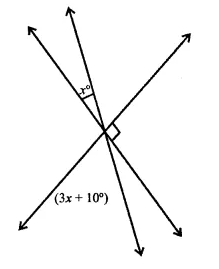Solution:
∠1 = 3x+ 10 (Vertically opposite angles)
But x + ∠1 + ∠2 = 180°⇒ x + 3x + 10° + 90° = 180°
⇒ 4x = 180° – 10° – 90° = 80°
x = $$\frac { { 80 }^{ \circ } }{ 4 }$$ = 20   (c)

Question 9.
In the figure, which of the following statements must be true?
(i) a + b = d + c
(ii) a + c + e = 180°
(iii) b + f= c + e
(a) (i) only
(b) (ii) only
(c) (iii) only
(d) (ii) and (iii) onlySolution:
In the figure,
(i) a + b = d + c
a° = d°
b° = e°
c°= f°
(ii) a + b + e = 180°
a + e + c = 180°
⇒ a + c + e = 180°
(iii) b + f= e + c
∴ (ii) and (iii) are true statements (d)

Question 10.
If two interior angles on the same side of a transversal intersecting two parallel lines are in the ratio 2:3, then the measure of the larger angle is
(a) 54°
(b) 120°
(c) 108°
(d) 136°
Solution:
In figure, l || m and p is transversal= $$\frac { 3 }{ 5 }$$ x 180° = 108° (c)

Question 11.
In the figure, if AB || CD, then the value of x is
(a) 20°
(b) 30°
(c) 45°
(d) 60°Solution:
In the figure, AB || CD,
and / is transversal
∠1 = x (Vertically opposite angles)
and 120° + x + ∠1 = 180° (Co-interior angles)Question 12.
Two lines AB and CD intersect at O. If ∠AOC + ∠COB + ∠BOD = 270°, then ∠AOC =
(a) 70°
(b) 80°
(c) 90°
(d) 180°
Solution:
Two lines AB and CD intersect at O∠AOC + ∠COB + ∠BOD = 270° …(i)
But ∠AOC + ∠COB + ∠BOD + ∠DOA = 360° …(ii)
Subtracting (i) from (ii),
∠DOA = 360° – 270° = 90°
But ∠DOA + ∠AOC = 180°
∴ ∠AOC = 180° – 90° = 90° (c)

Question 13.
In the figure, PQ || RS, ∠AEF = 95°, ∠BHS = 110° and ∠ABC = x°. Then the value of x is
(a) 15°
(b) 25°
(c) 70°
(d) 35°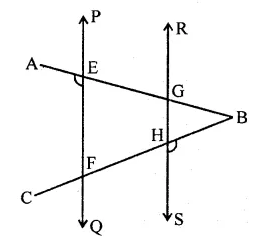Solution:
In the figure,PQ || RS, ∠AEF = 95°
∠BHS = 110°, ∠ABC = x
∵ PQ || RS,
∴ ∠AEF = ∠1 = 95° (Corresponding anlges)
But ∠1 + ∠2 = 180° (Linear pair)
⇒ ∠2 = 180° – ∠1 = 180° – 95° = 85°
In ∆AGH,
Ext. ∠BHS = ∠2 +x
⇒ 110° = 85° + x
⇒ x= 110°-85° = 25° (b)

Question 14.
In the figure, if l1 || l2, what is the value of x?
(a) 90°
(b) 85°
(c) 75°
(d) 70°Solution:
In the figure,∠1 = 58° (Vertically opposite angles)
Similarly, ∠2 = 37°
∵ l1 || l2, EF is transversal
∠GEF + EFD = 180° (Co-interior angles)
⇒ ∠2 + ∠l +x = 180°
⇒ 37° + 58° + x = 180°
⇒ 95° + x= 180°
x = 180°-95° = 85° (b)

Question 15.
In the figure, if l1 || l2, what is x + y in terms of w and z?
(a) 180-w + z
(b) 180° + w- z
(c) 180 -w- z
(d) 180 + w + zSolution:
In the figure, l1 || l2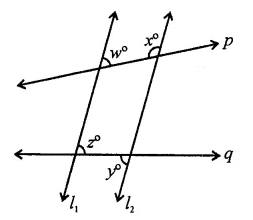p and q are transversals
∴ w + x = 180° ⇒ x = 180° – w (Co-interior angle)
z = y (Alternate angles)
∴ x + y = 180° – w + z (a)

Question 16.
In the figure, if l1 || l2, what is the value of y?
(a) 100
(b) 120
(c) 135
(d) 150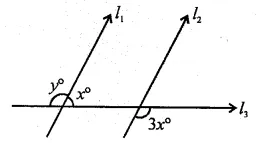Solution:
In the figure, l1 || l2 and l3 is the transversalQuestion 17.
In the figure, if l1 || l2 and l3 || l4 what is y in terms of x?
(a) 90 + x
(b) 90 + 2x
(c) 90 – $$\frac { x }{ 2 }$$
(d) 90 – 2x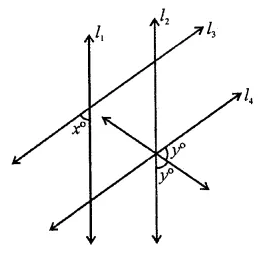Solution:
In the figure,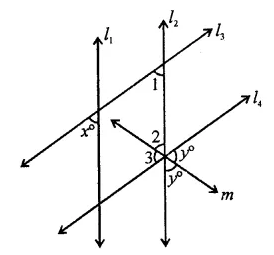l1 || l2 and l3 || l4 and m is the angle bisector
∴ ∠2 = ∠3 = y
∵ l1 || l2
∠1 = x (Corresponding angles)
∵ l3 || l4
∴ ∠1 + (∠2 + ∠3) = 180° (Co-interior angles)
⇒ x + 2y= 180°
⇒ 2y= 180°-x
⇒ y = $$\frac { { 540 }^{ \circ }-x }{ 4 }$$
= 90° – $$\frac { x }{ 2 }$$ (c)

Question 18.
In the figure, if 11| m, what is the value of x?
(a) 60
(b) 50
(c) 45
d) 30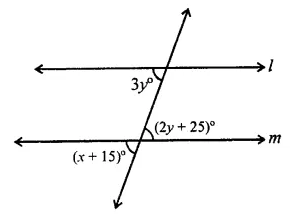Solution:
In the figure, l || m and n is the transversal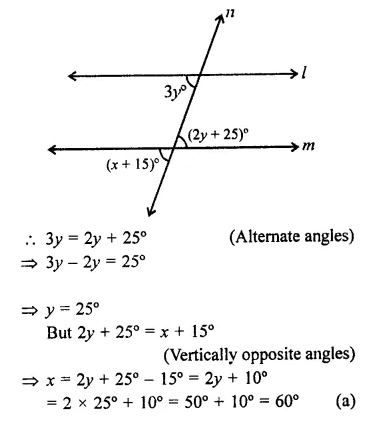⇒ y = 25°
But 2y + 25° = x+ 15°
(Vertically opposite angles) ⇒ x = 2y + 25° – 15° = 2y+ 10°
= 2 x 25°+10° = 50°+10° = 60° (a)

Question 19.
In the figure, if AB || HF and DE || FG, then the measure of ∠FDE is
(a) 108°
(b) 80°
(c) 100°
(d) 90°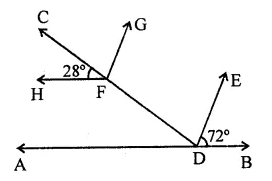Solution:
In the figure,
AB || HF, DE || FG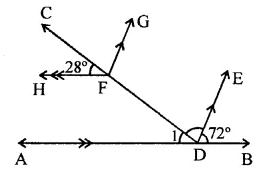∴ HF || AB
∠1 =28° (Corresponding angles)
But ∠1 + ∠FDE + 72° – 180° (Angles of a straight line)
⇒ 28° + ∠FDE + 72° = 180°
⇒ ∠FDE + 100° = 180°
⇒ ∠FDE = 180° – 100 = 80° (b)

Question 20.
In the figure, if lines l and m are parallel, then x =
(a) 20°
(b) 45°
(c) 65°
(d) 85°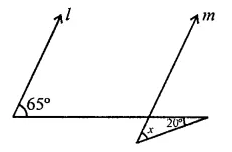Solution:
In the figure, l || m∴ ∠1 =65° (Corresponding angles)
In ∆BCD,
Ext. ∠1 = x + 20°
⇒ 65° = x + 20°
⇒ x = 65° – 20°
⇒ x = 45° (b)

Question 21.
In the figure, if AB || CD, then x =
(a) 100°
(b) 105°
(c) 110°
(d) 115°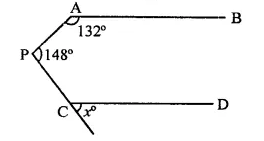Solution:
In the figure, AB || CD
Through P, draw PQ || AB or CD∠A + ∠1 = 180° (Co-interior angles)
⇒ 132° + ∠1 = 180°
⇒ ∠1 = 180°- 132° = 48°
∴ ∠2 = 148° – ∠1 = 148° – 48° = 100°
∵ DQ || CP
∴ ∠2 = x (Corresponding angles)
∴ x = 100° (a)

Question 22.
In tlie figure, if lines l and in are parallel lines, then x =
(a) 70°
(b) 100°
(c) 40°
(d) 30°Solution:
In the figure, l || m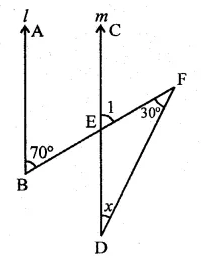∠l =70° (Corresponding angles)
In ∆DEF,
Ext. ∠l = x + 30°
⇒ 70° = x + 30°
⇒ x = 70° – 30° = 40° (c)

Question 23.
In the figure, if l || m, then x =
(a) 105°
(b) 65°
(c) 40°
(d) 25°Solution:
In the figure,
l || m and n is the transversal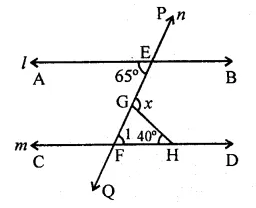∠1 = 65° (Alternate angles)
In ∆GHF,
Ext. x = ∠1 + 40° = 65° + 40°
⇒ x = 105°
∴ x = 105° (a)

Question 24.
In the figure, if lines l and m are parallel, then the value of x is
(a) 35°
(b) 55°
(c) 65°
(d) 75°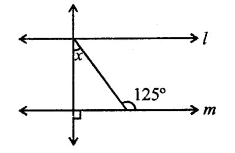Solution:
In the figure, l || m
and PQ is the transversal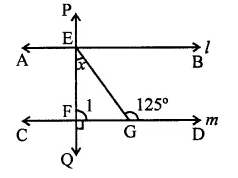∠1 = 90°
In ∆EFG,
Ext. ∠G = ∠E + ∠F
⇒ 125° = x + ∠1 = x + 90°
⇒ x = 125° – 90° = 35° (a)

Question 25.
Two complementary angles are such that two times the measure of one is equal to three times the measure of the other. The measure of the smaller angle is
(a) 45°
(b) 30°
(c) 36°
(d) none of these
Solution:
Let first angle = x
Then its complementary angle = 90° – x
∴ 2x = 3(90° – x)
⇒ 2x = 270° – 3x
⇒ 2x + 3x = 270°
⇒ 5x = 270°
⇒ x = $$\frac { { 270 }^{ \circ } }{ 5 }$$  = 54°
∴ second angle = 90° – 54° = 36°
∴ smaller angle = 36° (c)

Question 26.Solution: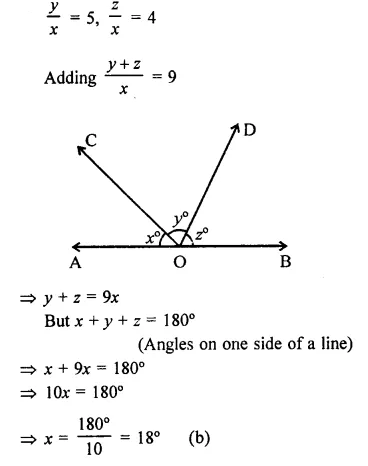Question 27.
In the figure, AB || CD || EF and GH || KL.
The measure of ∠HKL is
(a) 85°
(b) 135°
(c) 145°
(d) 215°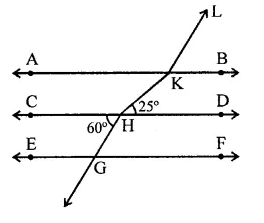Solution:
In the figure, AB || CD || EF and GH || KL and GH is product to meet AB in L.∵ AB || CD
∴ ∠1 = 25° (Alternate angle)
and GH || KL
∴ ∠4 = 60° (Corresponding angles)
∠5 = ∠4 = 60° (Vertically opposite angle)
∠5 + ∠2 = 180° (Co-interior anlges)
∴ ⇒ 60° + ∠2 = 180°
∠2 = 180° – 60° = 120°
Now ∠HKL = ∠1 + ∠2 = 25° + 120°
= 145° (c)

Question 28.
AB and CD are two parallel lines. PQ cuts AB and CD at E and F respectively. EL is the bisector of ∠FEB. If ∠LEB = 35°, then ∠CFQ will be
(a) 55°
(b) 70°
(c) 110°
(d) 130°
Solution:
AB || CD and PQ is the transversal EL is the bisector of ∠FEB and ∠LEB = 35°∴ ∠FEB = 2 x 35° = 70°
∵ AB || CD
∴ ∠FEB + ∠EFD = 180°
(Co-interior angles)
70° + ∠EFD = 180°
∴ ∠EFD = 180°-70°= 110°
But ∠CFQ = ∠EFD
(Vertically opposite angles)
∴ ∠CFQ =110° (c)

Question 29.
In the figure, if line segment AB is parallel to the line segment CD, what is the value of y?
(a) 12
(b) 15
(c) 18
(d) 20Solution:
In the figure, AB || CD
BD is transversal
∴ ∠ABD + ∠BDC = 180° (Co-interior angles)
⇒y + 2y+y + 5y = 180°
⇒ 9y = 180° ⇒ y = $$\frac { { 180 }^{ \circ } }{ 9 }$$  = 20° (d)

Question 30.
In the figure, if CP || DQ, then the measure of x is
(a) 130°
(b) 105°
(c) 175°
(d) 125°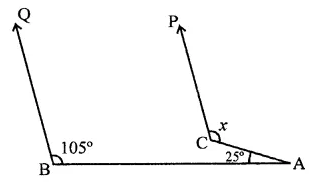Solution:
In the figure, CP || DQ
BA is transversal
Produce PC to meet BA at D∵ QB || PD
∴ ∠D = 105° (Corresponding angles)